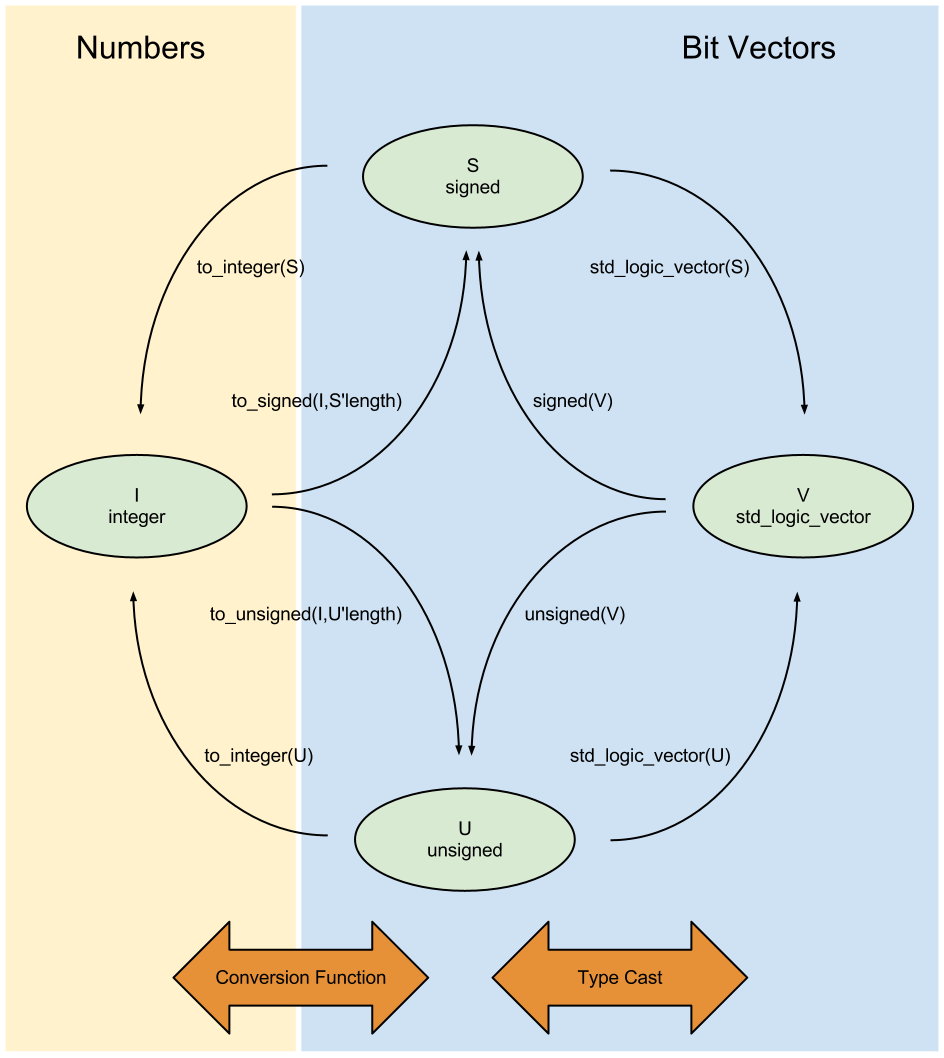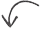# VHDL Type Conversion

Posted by in on 2-10-13

Any given VHDL FPGA design may have multiple VHDL types being used. The most common VHDL types used in synthesizable VHDL code are std_logic, std_logic_vector, signed, unsigned, and integer. Because VHDL is a strongly-typed language, most often differing types cannot be used in the same expression. In cases where you can directly combine two types into one expression, you are really leaving it up to the compiler or synthesis tool to determine how the expression should behave, which is a dangerous thing to do.

1. Type casting and conversion functions.
2. The importance of using the appropriate type.
3. Common uses and examples.

VHDL Type Cast and Conversion Functions

The picture below illustrates how to convert between the most common VHDL types.Type casting is used to move between the std_logic_vector type and the signed and unsigned types.

[cc lang=”vhdl” noborder=”true” tab_size=”4″ lines=”-1″ width=”600″ escaped=”true”]
–signal definitions
signal slv : std_logic_vector(7 downto 0);
signal s : signed(7 downto 0);
signal us : unsigned(7 downto 0);

–FROM std_logic_vector TO signed/unsigned
sgn <= signed(slv);
usgn <= unsigned(slv);

— FROM signed/unsigned TO std_logic_vector
svl <= std_logic_vector(sgn);
svl <= std_logic_vector(usgn);
[/cc]

Functions are used to move between signed and unsigned types and the integer type.

[cc lang=”vhdl” noborder=”true” tab_size=”4″ lines=”-1″ width=”600″ escaped=”true”]
–signal definitions
signal i : integer;
signal sgn : signed(7 downto 0);
signal usgn : unsigned(7 downto 0);

–FROM integer TO signed/unsigned
sgn <= to_signed(i,8);
usgn <= to_unsigned(i,8);

— FROM signed/unsigned TO integer
i <= to_integer(sgn);
i <= to_integer(usgn);
[/cc]

An easy way to remember when to use function or type cast is to remember that both the std_logic_vector and signed/unsigned types both are defined with a specific bit width, while integers do not define a bit width.

A type cast between std_logic_vector and signed/unsigned can be used as long as the origin and destination signals have the same bit width. Integers do not have a set bit width, which is why the conversion function from integer to signed/unsigned includes a specification of the intended bit width.

As a side note on integers:

Notice that there is no direct conversion path between the std_logic_vector type and the integer type. Integer types do not have a set width, unlike signed, unsigned, and std_logic_vector types. To convert between integer and std_logic_vector types, you must first convert to signed or unsigned.

If you do not restrict the range when defining an integer, the compiler will assume a 32-bit width. Depending on your synthesis tool and its settings, the default bit width of 32 may or may not be optimized out to the appropriate bit width.

Using the Correct VHDL Type

Although it’s possible to perform many math functions using the std_logic_vector type, doing that is unnecessarily difficult in digital signal processing (DSP) designs. Use the signed and unsigned types to keep track of your precision and sign type. After all, why use VHDL if you’re not going to harness the power of the strong typing?

Don’t leave it up to the compiler or synthesis tool to figure out what operation you want to perform or what an expression result should be!

Common Uses and Examples

Some common examples of situations needing to use type casting or conversion are in mathematical expressions. Another very common use is when you want to use a counter value as in index into an array. There are many more uses, but we’ll just take a look at these two.

Take a look at what happens when we subtract two unsigned numbers:

[cc lang=”vhdl” noborder=”true” tab_size=”4″ lines=”-1″ width=”600″ escaped=”true”]
signal sum_u : unsigned(11 downto 0);
signal sum_s : signed(11 downto 0);
constant a : unsigned(3 downto 0) := X”A”;

sum_u <= counter_fr + (X”00″ & a);
sum_s <= signed(counter_fr) + signed(X”00″ & a);

[/cc]

Depending on whether the result is defined as signed or unsigned, the results are drastically different.  When counter_fr holds the value 1000 (binary), sum_u=18 and sum_s=2.  That’s because the binary value 1000 is 8 unsigned and -8 two’s complement signed.

Another common use is converting a std_logic_vector or unsigned type to an integer so that it can be used as an array index. Arrays can only be indexed with integers.

[cc lang=”vhdl” noborder=”true” tab_size=”4″ lines=”-1″ width=”600″ escaped=”true”]
constant vec : std_logic_vector(15 downto 0);
signal count : std_logic_vector(3 downto 0);
signal element : std_logic_vector(0 downto 0);

element <= vec(to_integer(unsigned(count),4));
[/cc]

Happy coding!

We want to hear from you!  Do you have a comment, question, or suggestion?  Feel free to drop us an email or post a comment.

## Related content:Did you enjoy this article?Share
the
Love